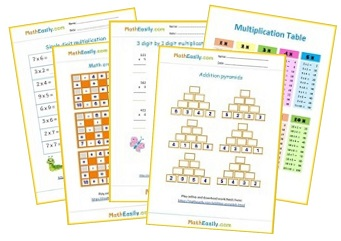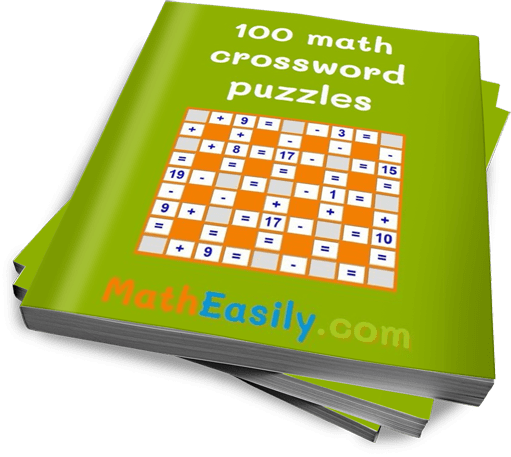﻿ MathEasily.com | Free online math games and worksheets

# MathEasily.com

is your resource of free online math games, activities and worksheets.
Learn addition, subtraction, multiplication, division and more.Free Math Worksheets

## Kindergarten Math

Addition with pictures, number lines, worksheets and puzzles for Preschoolers.
Start here

Start here

Number puzzles, multiplication, math worksheets etc. for the Second Grade.
Start here

Basics of multiplication, math games and worksheets for the Third Grade Math students.
Start here

Math games and worksheets for 4th Grade Math students. Learn multiplication, column subtraction and much more.
Start here

Math worksheets and games for 5th Grade Students. Practice two digit and three digit multiplication.
Start hereWho said learning math can't be exciting?

Have you ever tried any math crossword puzzle?

So if you are a parent or teacher, math puzzles are a great tool for helping kids to learn math easily.

## Enjoy our math learning games for kids

Learn addition, subtraction, multiplication and division.